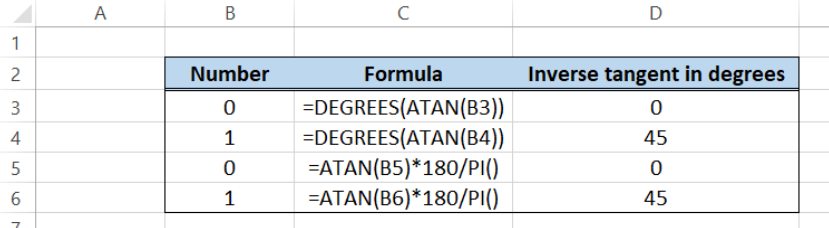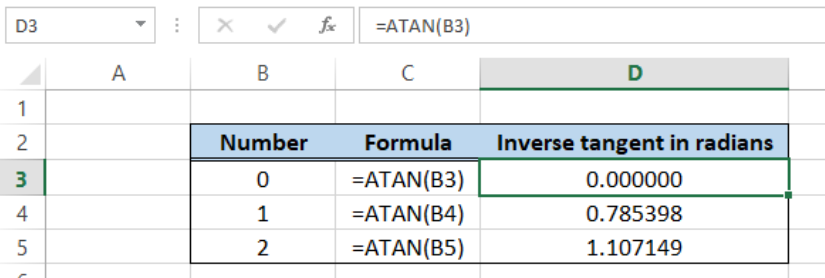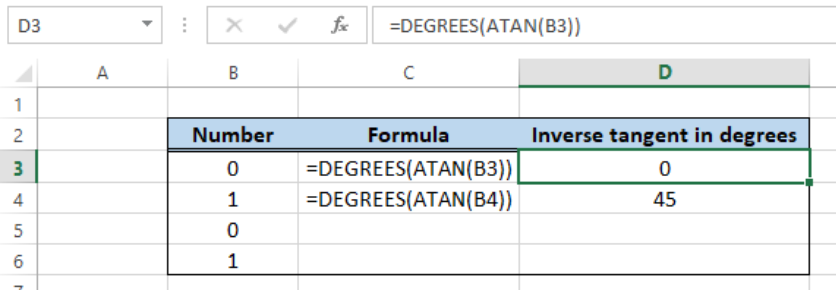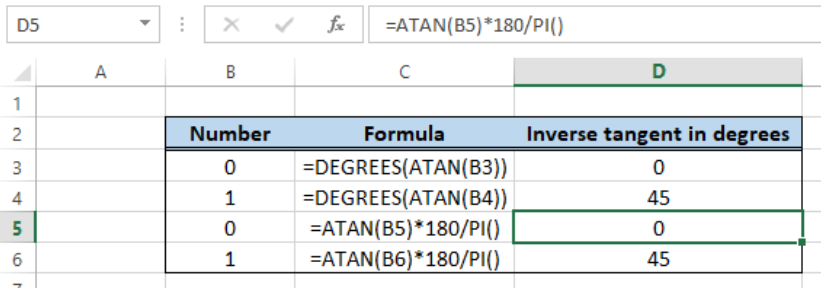Get instant live expert help with Excel or Google Sheets“My Excelchat expert helped me in less than 20 minutes, saving me what would have been 5 hours of work!”

#### Post your problem and you’ll get expert help in seconds.

Your message must be at least 40 characters
Our professional experts are available now. Your privacy is guaranteed.

# Inverse tangent in Excel – Excelchat

Trigonometry, as one of the difficult branches of Mathematics, has been feared by most students  because of the challenging laws and various trigonometric functions. One of the inverse trigonometric functions is the inverse tangent or arctangent.

The inverse tangent of a number is the angle in radians, whose tangent is the specified number.  The resulting angle ranges from -pi/2 to pi/2.  Fortunately, Excel provides us a way to calculate the inverse tangent of a number using the ATAN function.Figure 1.  Final result:  Inverse tangent

## Syntax of ATAN

`=ATAN(number)`

• where number is the tangent of the angle we want to obtain

## Calculate the arctan in radians

The inverse tangent or arctan of a number can be calculated in a straightforward manner.  Given a number in B3, we enter the formula in D3:

`=ATAN(B3)`Figure 2.  Output: Calculate inverse tangent using ATAN

Column D shows the resulting angles in radians corresponding to the arctan of numbers 0, 1 and 2.

## Calculate the arctan in degrees

To display the inverse tangent in degrees rather than radians, we can use the DEGREES function or multiply the angle by the conversion factor 180/PI().

Using the DEGREES function, enter these formulas in D3 and D4:

In D3: `=DEGREES(ATAN(B3)) `

In D4: `=DEGREES(ATAN(B4))`Figure 3.  Output: Arctan using ATAN and DEGREES

The resulting angle is 0 degrees for D3 and 45 degrees for D4.

By using the conversion factor, we enter these formulas in D5 and D6:

In D5: `=ATAN(B5)*180/PI()`

In D6: `=ATAN(B6)*180/PI()`Figure 4.  Output: Arctan in degrees

The resulting angles in degrees in D5 and D6 are the same as in D3 and D4.

## Instant Connection to an Excel Expert

Most of the time, the problem you will need to solve will be more complex than a simple application of a formula or function. If you want to save hours of research and frustration, try our live Excelchat service! Our Excel Experts are available 24/7 to answer any Excel question you may have. We guarantee a connection within 30 seconds and a customized solution within 20 minutes.